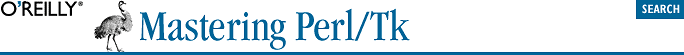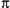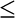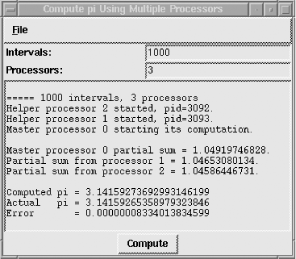home | O'Reilly's CD bookshelfs | FreeBSD | Linux | Cisco | Cisco Exam## 20.2. Computingwith Parallel Message Passing

Scientists demand the most powerful computing machinery available, whether they model global atmospheric conditions, decipher the human genome, study viscous fluid dynamics, visualize plasmas in 3 dimensions, or vibrate strings in 14. Besides tons of disk space (perhaps a terabyte for /tmp alone) and tremendous I/O and memory bandwidth, they want raw CPU power. Since no single CPU will ever suffice, scientists work with vector and massively parallel computers.

In parallel programming, we try to divide the work of a sequential program into portions so that many processors can work on the problem simultaneously. For example, this code computesby integrating the function 4 / (1 + x2) in the range -.5x < .5:

 This is a Perl version of the C example from the public domain MPICH distribution, available at http://www-unix.mcs.anl.gov/mpi/mpich/download.html. Notice two optimizations: the factor \$h is turned into multiplication because it's faster than division; likewise, multiplying \$x by itself is faster than exponentiation.

```my \$intervals = 1_000;
my \$h = 1.0 / \$intervals;
my \$sum = 0.0;

for(my \$i = 1; \$i <= \$intervals; \$i++) {
my \$x = \$h * (\$i - 0.5);
\$sum += 4.0 / (1.0 + \$x*\$x);
}

my \$pi = \$h * \$sum;```

The variable \$intervals defines the granularity of the summation and, hence, the accuracy of the computation. To get a good result, the interval must be very finely divided, which, of course, increases the program's running time. But suppose we parcel out the work to two processors, with each one integrating only half of the curve? If we then add the two partial sums, the value ofis the same, but the computational wall-clock time is halved. In fact, putting 10 processors to work completes the job an order of magnitude faster, because this problem scales well.

### 20.2.3. Tcl/Tk Master Processor Code

For brevity, we'll focus only on the three subroutines from pi.tcl that create the user interface (Figure 20-2) and handle the send/receive transactions.

create_interface places the MainWindow on the display and initializes its title and icon name. It then creates the MainWindow's menubar and adds the File menubutton cascade with a Quit menu item. With the exception of having to explicitly define the cascade menu, we do exactly this in Perl/Tk. Underneath the menubar are, essentially, two labeled Entry widgets, which are created from scratch by packing Label and Entry widgets side-by-side in container frames. (We'd use LabEntry widgets in Perl/Tk.) Finally, it packs a Text widget that displays results and a Compute button that initiates a computation.

```proc create_interface {} {

wm geometry . "+100+100"
wm title . "Compute pi Using Multiple Processors"
wm iconname . "pi"

.mb.file add command -label Quit -command {destroy .}

# Frame to hold itervals label and entry widgets.

frame .f
pack .f -fill x
label .f.s -text "Intervals: "
entry .f.e -width 25 -textvariable intervals
pack .f.s -side left
pack .f.e -side right

# Frame to hold number of processors label and entry widgets.

frame .f2
pack .f2 -fill x
label .f2.s -text "Processors: "
entry .f2.e -width 25 -textvariable numprocs
pack .f2.s -side left
pack .f2.e -side right

# Text widget to hold results.

text .t -width 50 -height 14
pack .t -fill both -expand 1

button .c -text Compute -command {compute_pi}
pack .c

}; # end create_interface```
```proc start_helpers_calculating {} {

global helper intervals numprocs pids proc sums
global wishexe

set proc [expr \$numprocs - 1]
set y 420
while {\$proc > 0} {
set pids(\$proc) [exec \$wishexe -name pihelp\${proc} \
-geometry +100+\${y} < \$helper &]
status "Helper processor \$proc started, pid=\$pids(\$proc)."
incr y 50
incr proc [expr -1]
}
after 2000

set proc [expr \$numprocs - 1]
while {\$proc > 0} {
send pihelp\${proc} "set numprocs \$numprocs; \
set proc \$proc; set intervals \$intervals"
incr proc [expr -1]
}

}; # end start_helpers_calculating```

sum_reduce simulates a sum reduction, which is a message-passing operation that reads data from all helper processors and reduces the results to a single value by summing. It waits for each helper, in turn, to store its partial sum in the sums associative array and kills the helper afterward. Finally, it adds its own partial sum and returns the reduced result,.

```proc sum_reduce {} {

# Do a sum reduction.  Fetch all the partial sums computed
# by the helper processors, and then add them to our partial
# sum.  The result is an approximation of pi.

global helper intervals numprocs pids proc sums

set pi 0
for {set proc 1} {\$proc < \$numprocs} {incr proc} {
tkwait variable sums(\$proc)
status "Partial sum from processor \$proc = \$sums(\$proc)."
set pi [expr \$pi + \$sums(\$proc)]
exec kill \$pids(\$proc)
}
return [expr \$pi + \$sums(0)]

}; # end sum_reduce```

Figure 20-3 shows the outcome.#### Figure 20-3. An estimated value of pi, with error